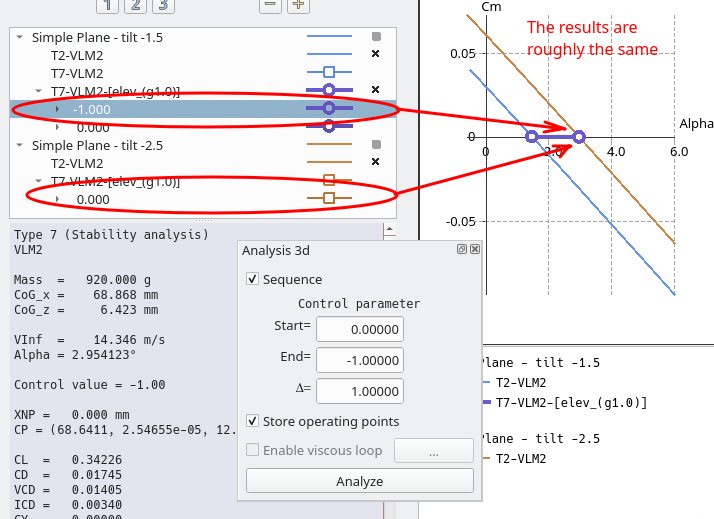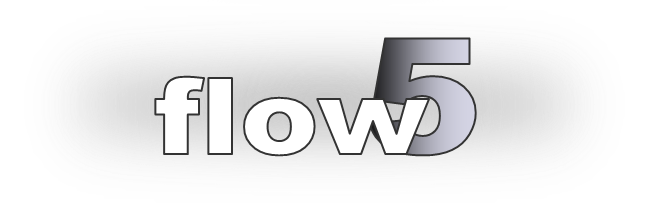# Stability analyses in flow5 Tutorial Updated March 25th, 2020

## Purpose

1. Summarize the steps to perform and post-process a stability analysis in flow5.
2. Describe the changes introduced in beta 07 to the calculation of control derivatives.

## Background theory

flow5 and xflr5 implement the theory of Etkin and Reid described in "Dynamics of flight - stability and control, 3rd edition, 1996."

A summary of the theory and the definition of the variables can be found here:
Lecture AC-1 Aircraft Dynamics
Lecture AC-2 Aircraft Longitudinal Dynamics
Lecture AC-3 Aircraft Lateral Dynamics

## Project file

The project files used for this tutorial and the corresponding AVL package can be downloaded here

## Step 1: Plane definition

### Define the Plane

As in previous versions of flow5 and xflr5, define the plane geometry and its inertia such that

1. the slope of the curve Cm = f(alpha) is negative → static stability
2. the lift coefficient is positive for the aoa such that Cm = 0 → the plane flies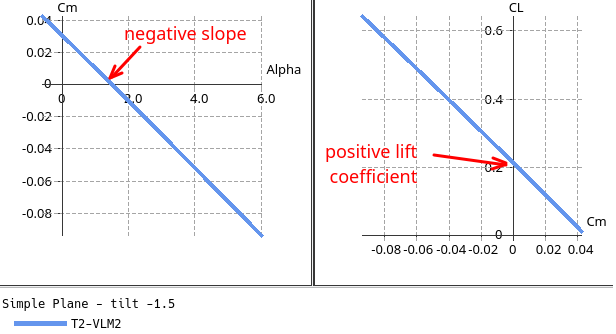### Define the control surfaces

The wing and flap angles are used as reference values for the sensitivity calculations.

#### Reference angular position θ0 of each wing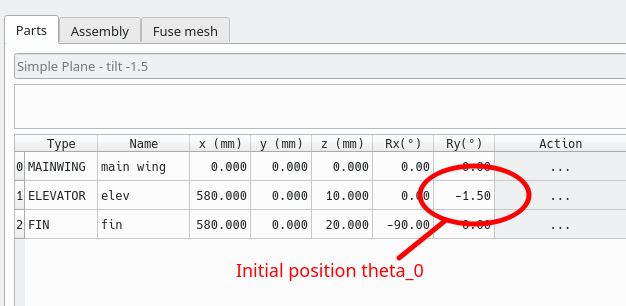#### Wing flaps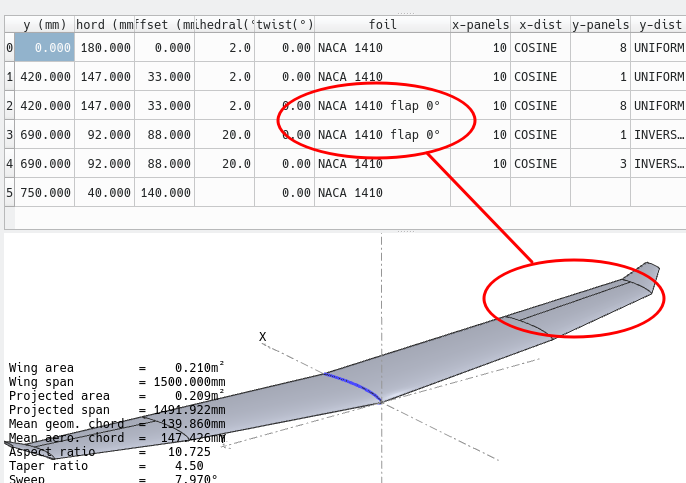The initial wing flap angle θ0 is defined by the foil flap angle.
To keep it simple, the recommendation is to set the flap angles to 0°.

## Step 2: Define and run a first stability analysis

The goal is to check that the analysis gives reasonable results and that the plane is sound.

De-activate the control surfaces by setting all the control coefficients and the gains to 0 for this first run.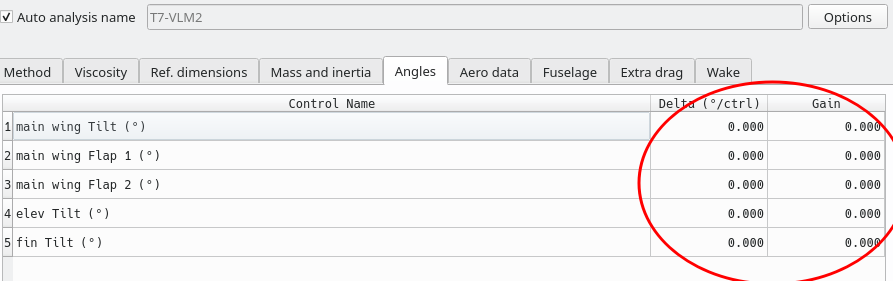Run the analysis for any value of the control parameter: since no active control surface has been activated, the value of the control parameter is not used.

Store the operating point.

If the analysis went well, an operating point will have been generated.

Select it to access the data in the properties box, e.g. the stability derivatives.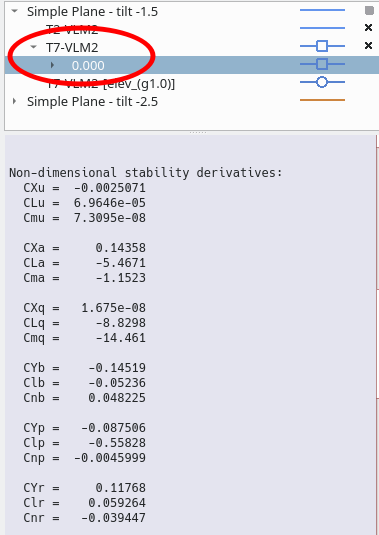### Root locus view

Check that the analysis produces the familiar conventional modes.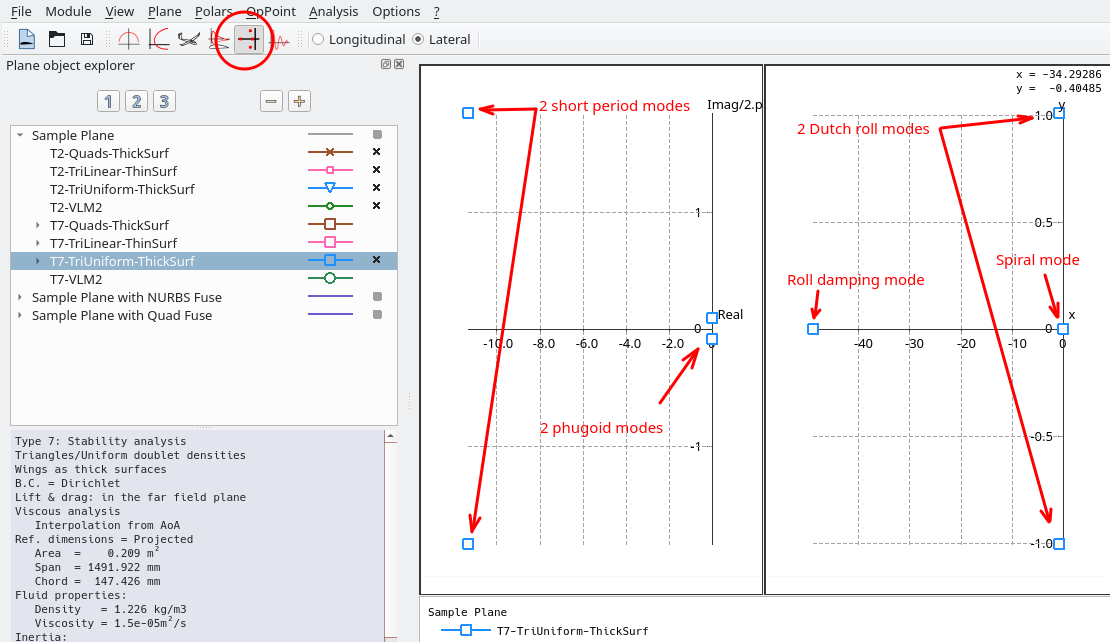To identify a mode in the root locus graphs, select it and activate "Highlight selection" in the graph's context menu or in the toolbox in the bottom right.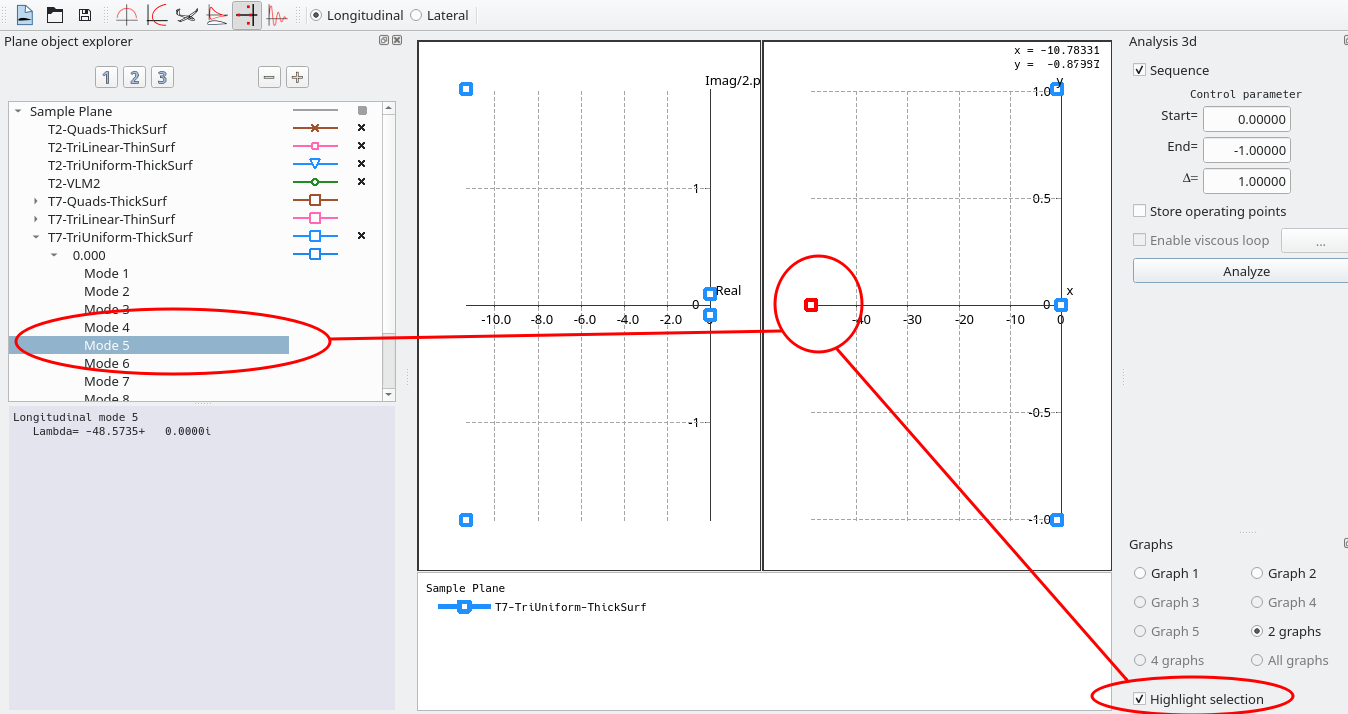### 3d view

Select a mode and animate the view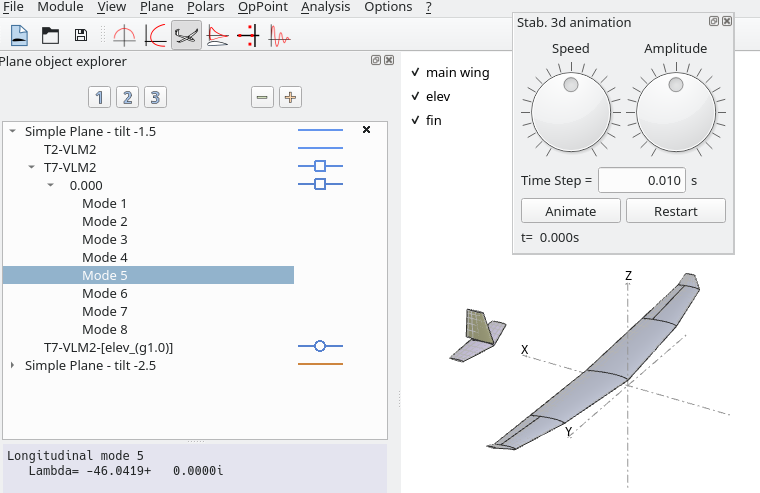### Time graphs view

Note: This post-processing module may not be as mature as the others – feedback and suggestions are welcome.

In the top toolbar, select the direction of interest - longitudinal or lateral.

Select the type 7 operating point.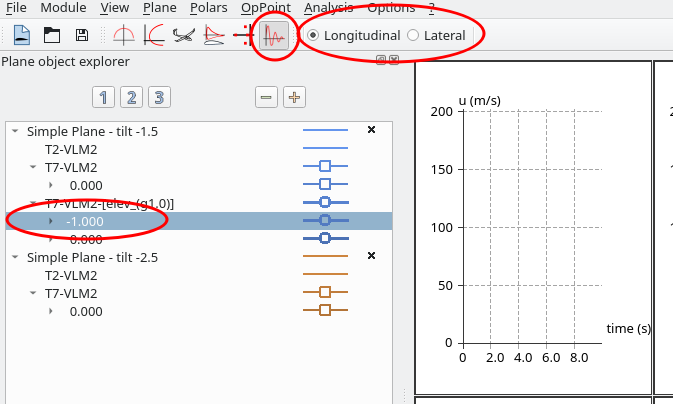There are 3 different types of response which can be plotted:

1. A perturbation to the stable flight conditions
2. A forced response to a control order – will be available at the next step when a control surface is defined and activated with a gain
3. A pure modal response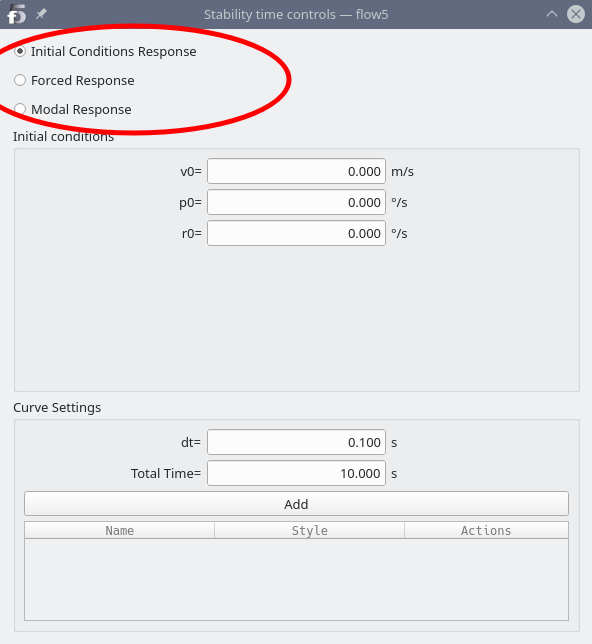Set the initial conditions to something other than zero values, or select a mode, then click "Add".
The curves will be plotted in the graphs.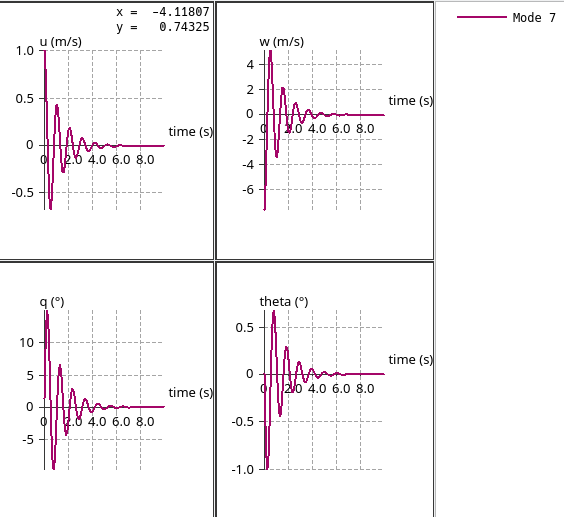## Step 3: Activate the control surfaces

The control parameters available in flow5 are:

1. the angular position of each wing around the y-axis
2. the flaps defined in each wing

In xflr5 and flow5 up to beta 06, the gain is used both to set the mean position of the control surface AND to calculate the control derivative. This creates an unfortunate confusion.

These settings are dissociated in flow5 v7.01 beta 07: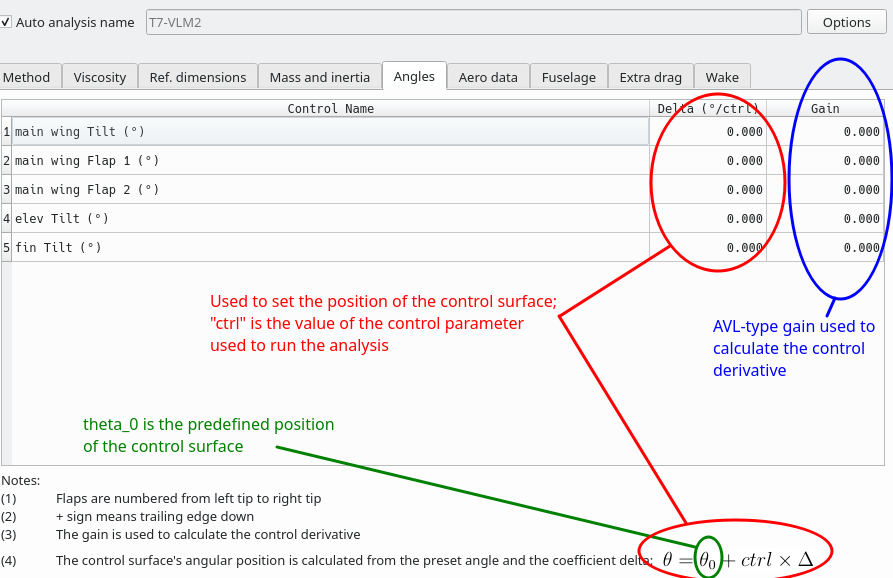Set the elevator's delta coefficient to 1 and its gain to 1 also.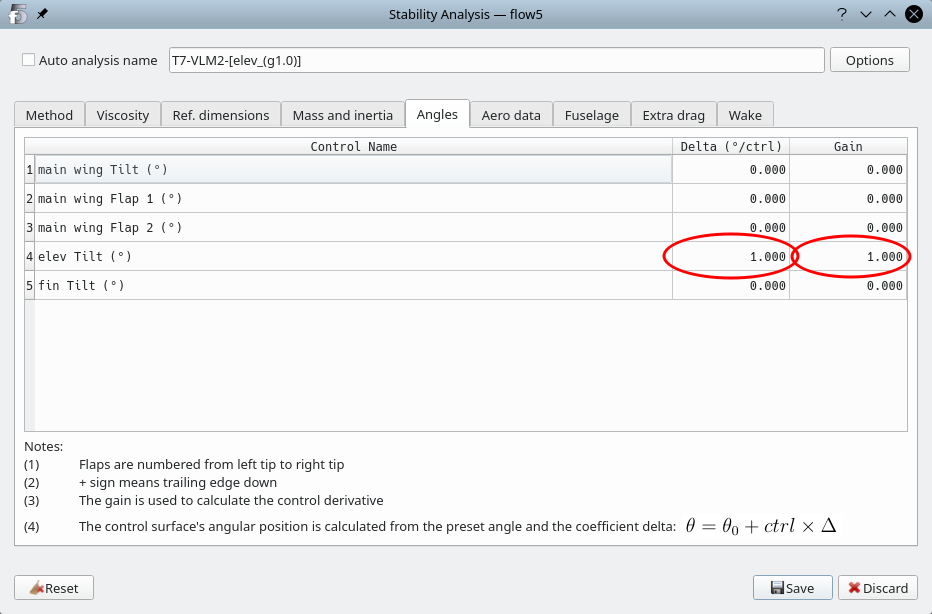Note that the delta coefficient and the gains do not need to be the same.
To simulate aileron differential, the delta coefficient would be set to 0, and the gains could be set for instance to +1 on the left wing's flap and to -2 on the right wing's flap.

Run an analysis for a given value of the control parameter.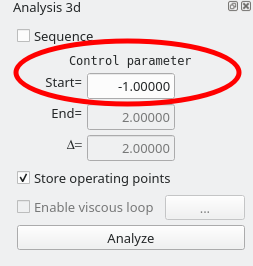In this case, the elevator will be set at:

-1.5°0 = the value defined in the plane) + [1 (the delta coefficient) x -1 (the ctrl parameter) ] = -2.5°

The analysis will produce values for the control derivatives: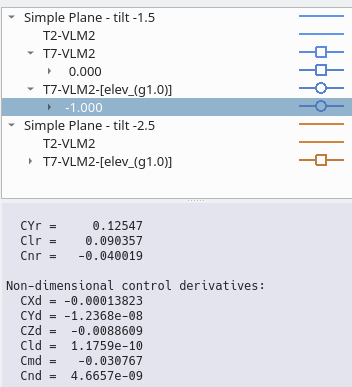### Response to actuation

Since a control surface is active, it is now possible to plot a forced response in the time graph view.

Use the spline to define the time-shape of the input order, then click “Add” to plot the response.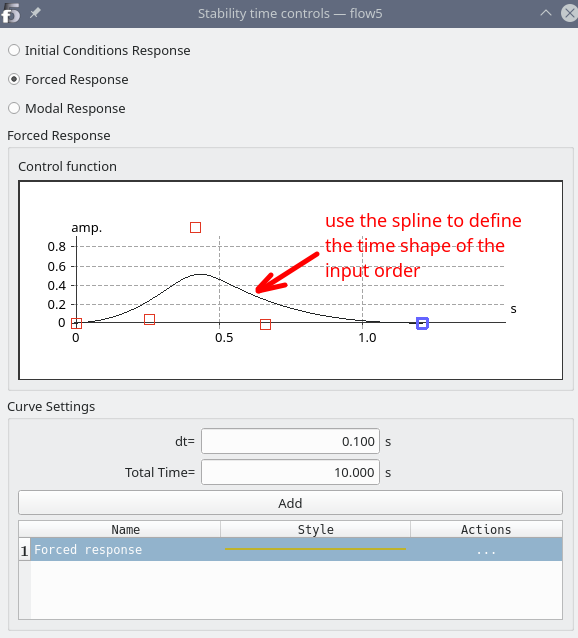### Design sensitivity

With an active control surface, the polar data can be plotted as a function of the control parameter.

Run the analysis for different values of the control parameter, then, In the polar graph view, plot the mode properties as a function of the control parameter, i.e. indirectly as a function of the position of the active control surfaces.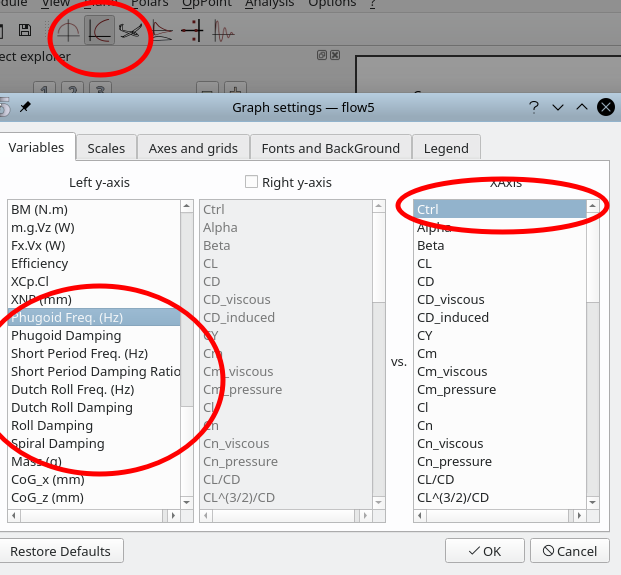## Step 4: Sanity check (optional)

The goal is to check that the previous analysis gives the same results as those obtained by running a stability analysis on a plane with an elevator positioned angularly at -2.5°.

Duplicate the plane, and set its elevator tilt to -2.5°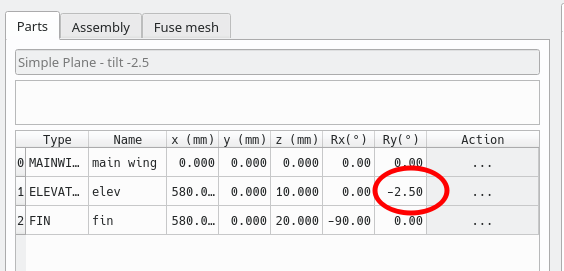Run a stability analysis with no active control surface, and generate the operating point.

The results should be the same as those of the plane with an elevator defined at -1.5° and an operating point generated for the ctrl parameter equal to -1.
The results are not exactly the same because the mesh panels are not constructed in the same way in each case.